# demos_source/code_TestShell.c File Reference

`#include "femtoos_code.h"`

Include dependency graph for code_TestShell.c: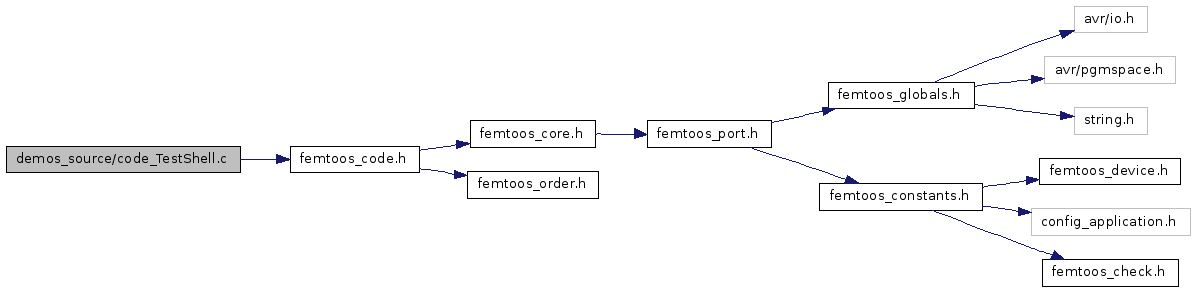Go to the source code of this file.

## Defines

#define defDemoRequestLedState   0x20
This file is solely for demonstration purposes.
#define defDemoRequestLedCycle   0x21
#define defDemoRequestLedSave   0x22
#define defDemoRequestLedStateOff   0x00
#define defDemoRequestLedStateOn   0x01
#define defDemoRequestLedStateFlash   0x02
#define defDemoRequestLedStateFreeze   0x04
#define defDemoRequestLedStateFollow   0x05
#define defDemoRequestLedStateUndef   0xFF
#define defDemoResponseLedOK   0xA0
#define defDemoResponseLedError   0xA1
#define Maxtry   9
#define SETBIT(Reg, Pos, val)   if (val) { (Reg|=(1<<Pos)); } else { (Reg&=~(1<<Pos)); }
#define GETBIT(Reg, Pos)   ((Reg>>Pos) & 0x01)
#define SetDebugLed(nr)   SETBIT(PORTB,(nr+4),false);
#define ClrDebugLed(nr)   SETBIT(PORTB,(nr+4),true);
#define BlockLength   16
#define ledOff(bits)   { PORTA |= (bits); }
#define ledOn(bits)   { PORTA &= ~(bits); }
#define ledInv(bits)   { PINA = (bits); }
#define getLed(bits)   (PORTA & (bits))

## Typedefs

typedef Tuint08 bit

## Functions

static void initBus (void)
static void startCommunication (void)
static bit getSCLin ()
static bit getSDA ()
static bit getACK ()
static void setSCLout (bit b)
static void setSDA (bit b)
static void setACK (bit b)
static void propDelay ()
static void calcCRC (Tbyte b)
static void clearCRC ()
static Tbyte getCRC ()
static Tbool checkCRC (Tbyte b)
static void initPins ()
static void writeByte (Tbyte b)
static void startWrite ()
static void WriteQueuPipe (Tchar c)
static void SendID ()
static void SendTickCount ()
static void SendUptime ()
static void SendProcessList ()
static void SendSingleResponse (Tuint08 uiResponse)
static Tuint08 getSingleValue ()
static void HandleReboot ()
static void HandleRecreate ()
static void HandleCommand (void(*pCommand)(Tuint08 uiTN))
static void HandlePriority ()
static void HandleDump ()
static void HandleStore ()
static void HandleRecall ()
static void HandleTest ()
static void ExeLedState ()
static void ExeLedCycle ()
static void ExeLedSave ()
static void ExecuteShell ()
static void clearQueus ()
void appBoot (void)
Prototype for the general initialization.
void appLoop_T4Bus (void)
void appLoop_Shell (void)
void appTick16 (void)
Tick hook called every tick rollover (65536 ticks).
static void wait (Tuint08 uiLed) __attribute__((noreturn))
void SIG_PIN_CHANGE (void)

## Variables

static Tbyte crcValue
static Tbool virgin
static Tbool prevMaster
static Tbool crcCheckResult
static Tuint08 uptime 
const Tchar FemtoOSid[] PROGMEM = SystemID
static Tuint08 volatile uiLedState 
static Tuint08 volatile uiPeriodOff 
static Tuint08 volatile uiPeriodOn 

## Define Documentation

 #define BlockLength   16

Definition at line 327 of file code_TestShell.c.

Referenced by HandleRecall(), and HandleStore().

 #define ClrDebugLed ( nr ) SETBIT(PORTB,(nr+4),true);

Definition at line 86 of file code_TestShell.c.

 #define defDemoRequestLedCycle   0x21

Definition at line 52 of file code_TestShell.c.

Referenced by ExecuteShell().

Definition at line 54 of file code_TestShell.c.

Referenced by ExecuteShell().

 #define defDemoRequestLedSave   0x22

Definition at line 53 of file code_TestShell.c.

Referenced by ExecuteShell().

 #define defDemoRequestLedState   0x20

This file is solely for demonstration purposes.

This example is made to demonstrate what all is possible with a device as small as the ATtiny861. It runs eight preemptive led blinkers that can be set to different states and blinker frequencies independently. There is one task running a 4 wire bus protocol (full handshake, variable speed, interruptible). The last task is a shell task through which you can talk to the device. Of course a terminal application is needed which generates the right commands and which understands the communication protocol. This application (on windows) is included. Besides controlling the activity of the leds, it also is possible to suspend and resume the task. Change priority of tasks, restart crashed tasks, reboot the system etc etc. Type 'help' to see all possibilities.

Extra hardware and external software is needed to run this example.

NOTE: This demo is not yet finished, and must still be virtualized to work on other hardware, and adapted so it can work with general terminals.

Definition at line 51 of file code_TestShell.c.

Referenced by ExecuteShell().

 #define defDemoRequestLedStateFlash   0x02

Definition at line 57 of file code_TestShell.c.

Referenced by appBoot(), and wait().

 #define defDemoRequestLedStateFollow   0x05

Definition at line 59 of file code_TestShell.c.

Referenced by ExeLedState(), and wait().

 #define defDemoRequestLedStateFreeze   0x04

Definition at line 58 of file code_TestShell.c.

Referenced by wait().

 #define defDemoRequestLedStateOff   0x00

Definition at line 55 of file code_TestShell.c.

Referenced by wait().

 #define defDemoRequestLedStateOn   0x01

Definition at line 56 of file code_TestShell.c.

Referenced by wait().

 #define defDemoRequestLedStateUndef   0xFF

Definition at line 60 of file code_TestShell.c.

 #define defDemoResponseLedError   0xA1

Definition at line 62 of file code_TestShell.c.

Referenced by ExeLedCycle(), and ExeLedState().

 #define defDemoResponseLedOK   0xA0

Definition at line 61 of file code_TestShell.c.

Referenced by ExeLedCycle(), and ExeLedState().

 #define GETBIT ( Reg, Pos ) ((Reg>>Pos) & 0x01)

Definition at line 83 of file code_TestShell.c.

Referenced by getACK(), getSCLin(), and getSDA().

 #define getLed ( bits ) (PORTA & (bits))

Definition at line 534 of file code_TestShell.c.

Referenced by wait().

 #define ledInv ( bits ) { PINA = (bits); }

Definition at line 533 of file code_TestShell.c.

 #define ledOff ( bits ) { PORTA |= (bits); }

Definition at line 531 of file code_TestShell.c.

Referenced by wait().

 #define ledOn ( bits ) { PORTA &= ~(bits); }

Definition at line 532 of file code_TestShell.c.

Referenced by wait().

 #define Maxtry   9

Definition at line 80 of file code_TestShell.c.

Referenced by startCommunication().

 #define SETBIT ( Reg, Pos, val ) if (val) { (Reg|=(1<

Definition at line 82 of file code_TestShell.c.

Referenced by setACK(), setSCLout(), and setSDA().

 #define SetDebugLed ( nr ) SETBIT(PORTB,(nr+4),false);

Definition at line 85 of file code_TestShell.c.

## Typedef Documentation

 typedef Tuint08 bit

Definition at line 67 of file code_TestShell.c.

## Function Documentation

 void appBoot ( void )

Prototype for the general initialization.

The Remember demonstration fills the file system with random numbers and calculates a (xor) hash on before hand. Then three worker tasks are started which read the files with concurrently and calculate hash's for each files. When all workers are done the main task combines the result and checks if it is equal to the value before. If so, a new round is started. Note that this demo wears out the EEPROM rapidly.

Definition at line 480 of file code_TestShell.c.

References defDemoRequestLedStateFlash, and Tuint08.

Definition at line 579 of file code_TestShell.c.

References wait().

Here is the call graph for this function:Definition at line 583 of file code_TestShell.c.

References wait().

Here is the call graph for this function:Definition at line 587 of file code_TestShell.c.

References wait().

Here is the call graph for this function:Definition at line 591 of file code_TestShell.c.

References wait().

Here is the call graph for this function:Definition at line 595 of file code_TestShell.c.

References wait().

Here is the call graph for this function:Definition at line 599 of file code_TestShell.c.

References wait().

Here is the call graph for this function: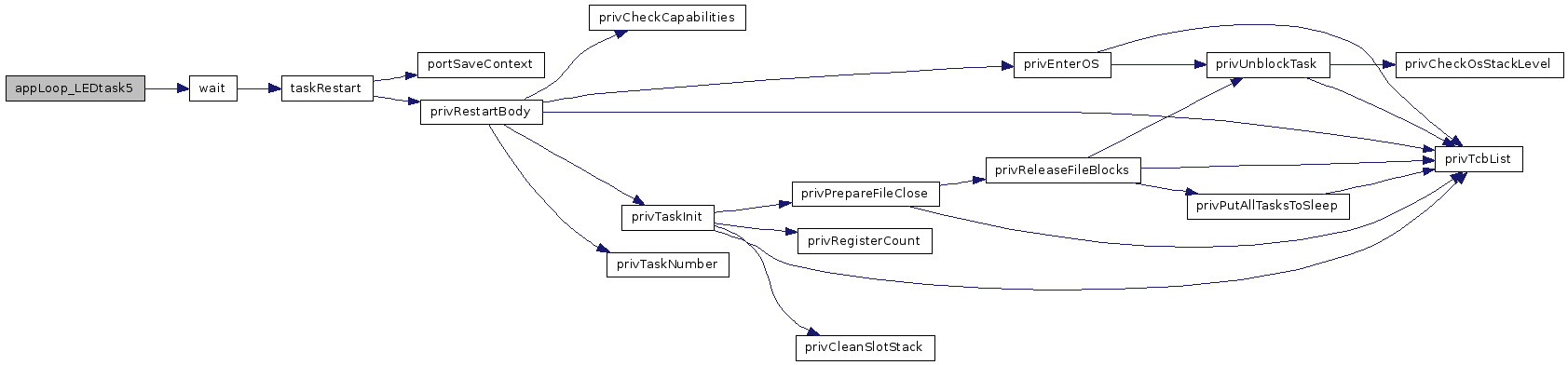Definition at line 603 of file code_TestShell.c.

References wait().

Here is the call graph for this function: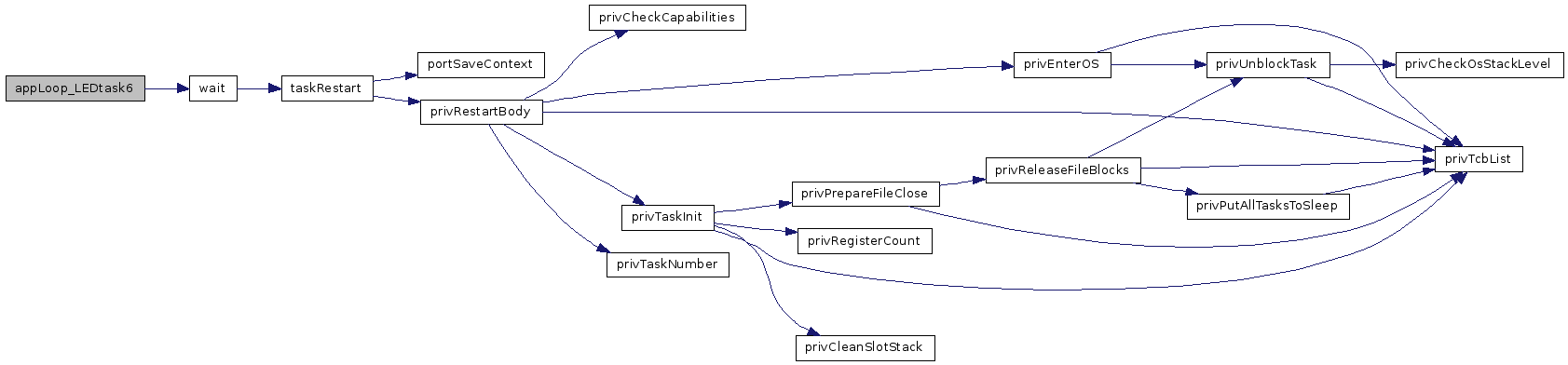Definition at line 607 of file code_TestShell.c.

References wait().

Here is the call graph for this function: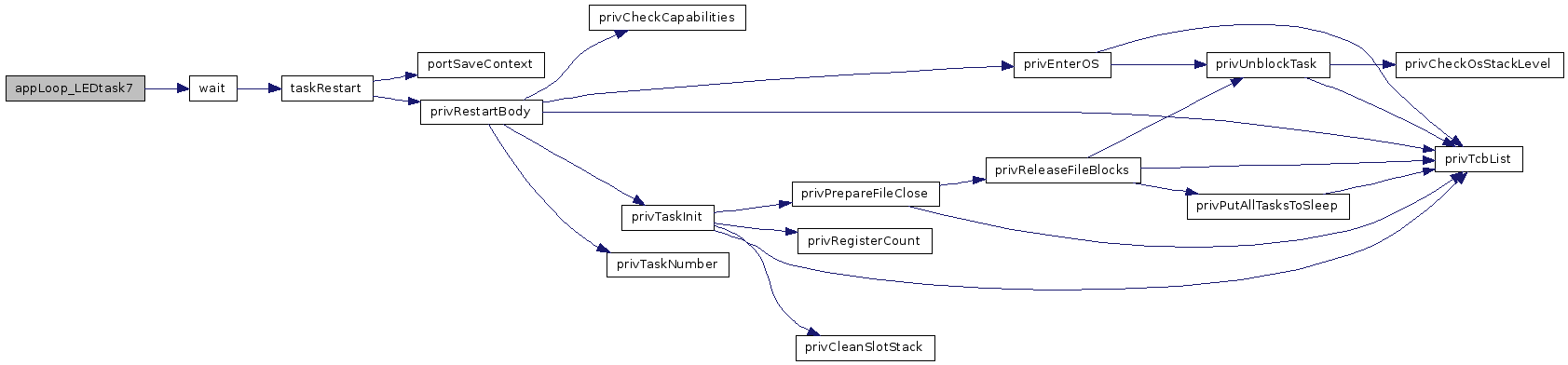void appLoop_Shell ( void )

Definition at line 515 of file code_TestShell.c.

Here is the call graph for this function:void appLoop_T4Bus ( void )

Definition at line 499 of file code_TestShell.c.

Here is the call graph for this function: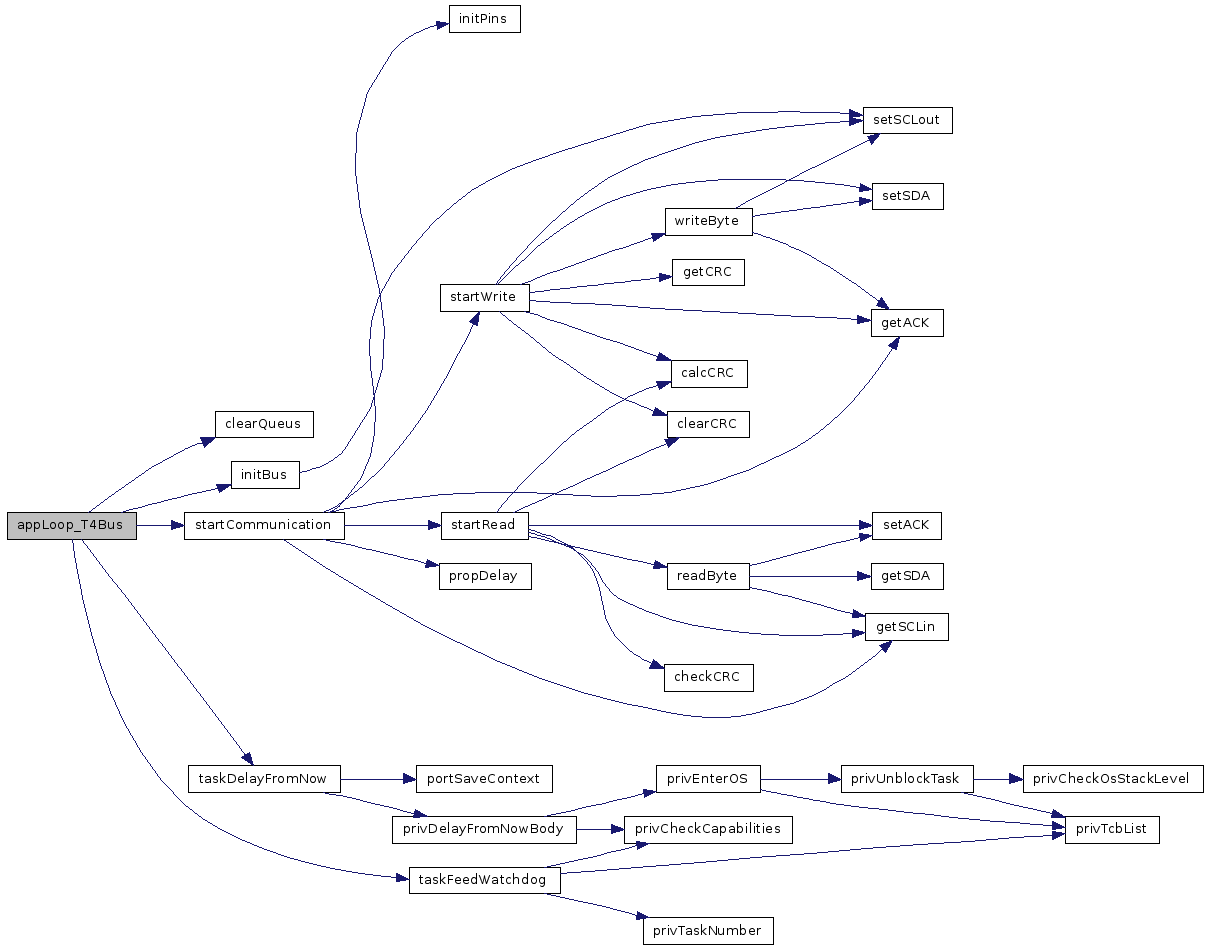void appTick16 ( void )

Tick hook called every tick rollover (65536 ticks).

Keep code inside short. API calls inside are not allowed, even the genXXX are not allowed. The tick counter may not be valid when called. This call is executed inside OS realm. In case of low power down, missed ticks are catched up, but may be deferred.

Definition at line 524 of file code_TestShell.c.

References uiDelayMode, uiDelayTimer, and uptime.

 static void calcCRC ( Tbyte b ) ` [static]`

Definition at line 109 of file code_TestShell.c.

Here is the caller graph for this function:static Tbool checkCRC ( Tbyte b ) ` [static]`

Definition at line 112 of file code_TestShell.c.

Here is the caller graph for this function: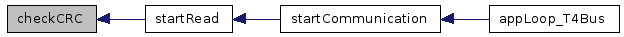static void clearCRC ( ) ` [static]`

Definition at line 110 of file code_TestShell.c.

Here is the caller graph for this function: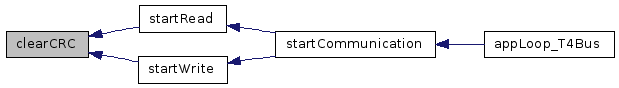static void clearQueus ( ) ` [static]`

Definition at line 470 of file code_TestShell.c.

Referenced by appLoop_Shell(), and appLoop_T4Bus().

Here is the caller graph for this function: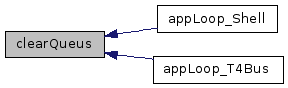static void ExecuteShell ( ) ` [static]`

Definition at line 447 of file code_TestShell.c.

Referenced by appLoop_Shell().

Here is the call graph for this function: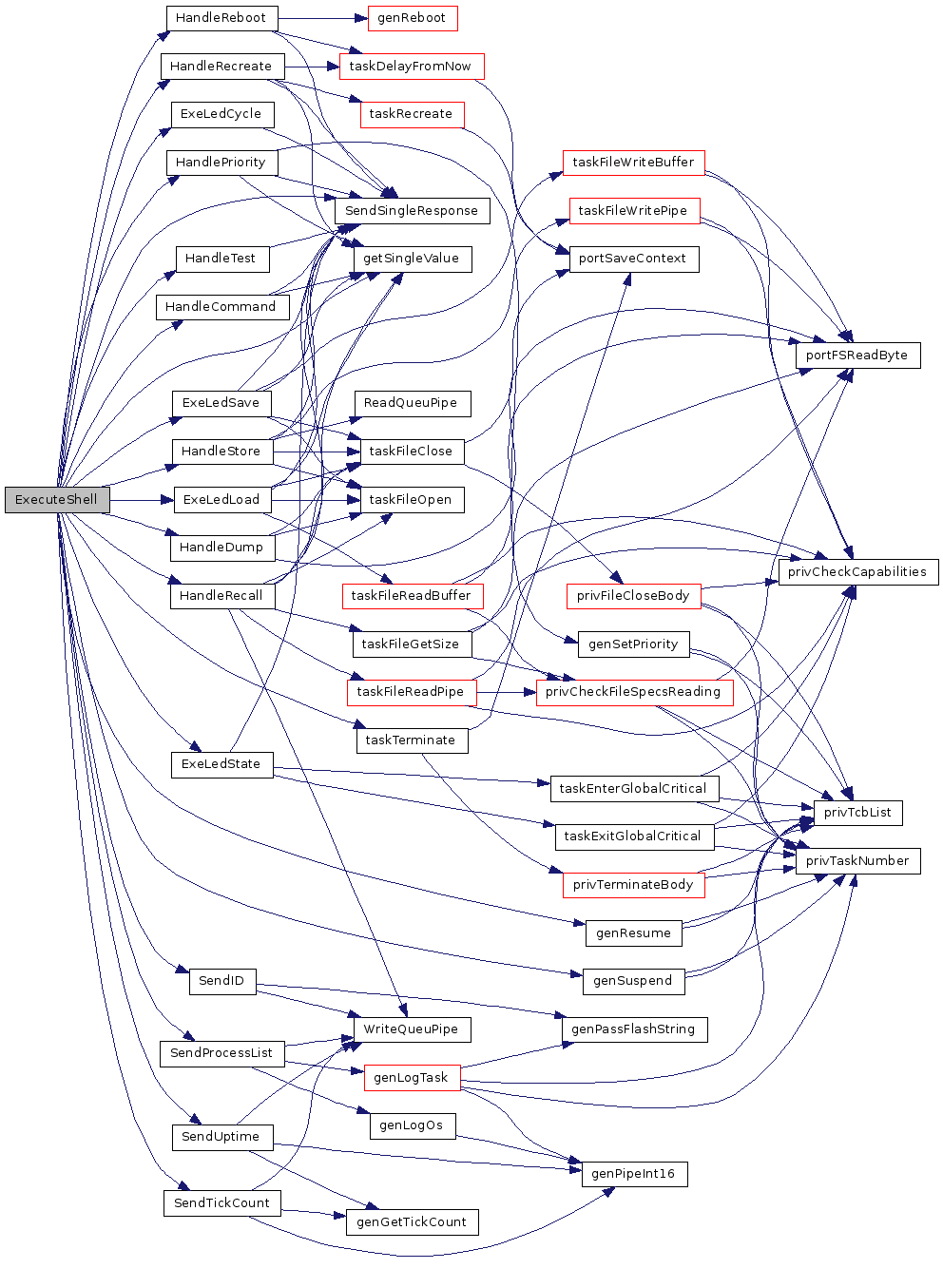Here is the caller graph for this function: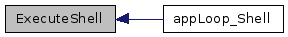static void ExeLedCycle ( ) ` [static]`

Definition at line 401 of file code_TestShell.c.

Referenced by ExecuteShell().

Here is the call graph for this function: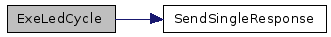Here is the caller graph for this function:static void ExeLedLoad ( ) ` [static]`

Definition at line 434 of file code_TestShell.c.

Referenced by ExecuteShell().

Here is the call graph for this function: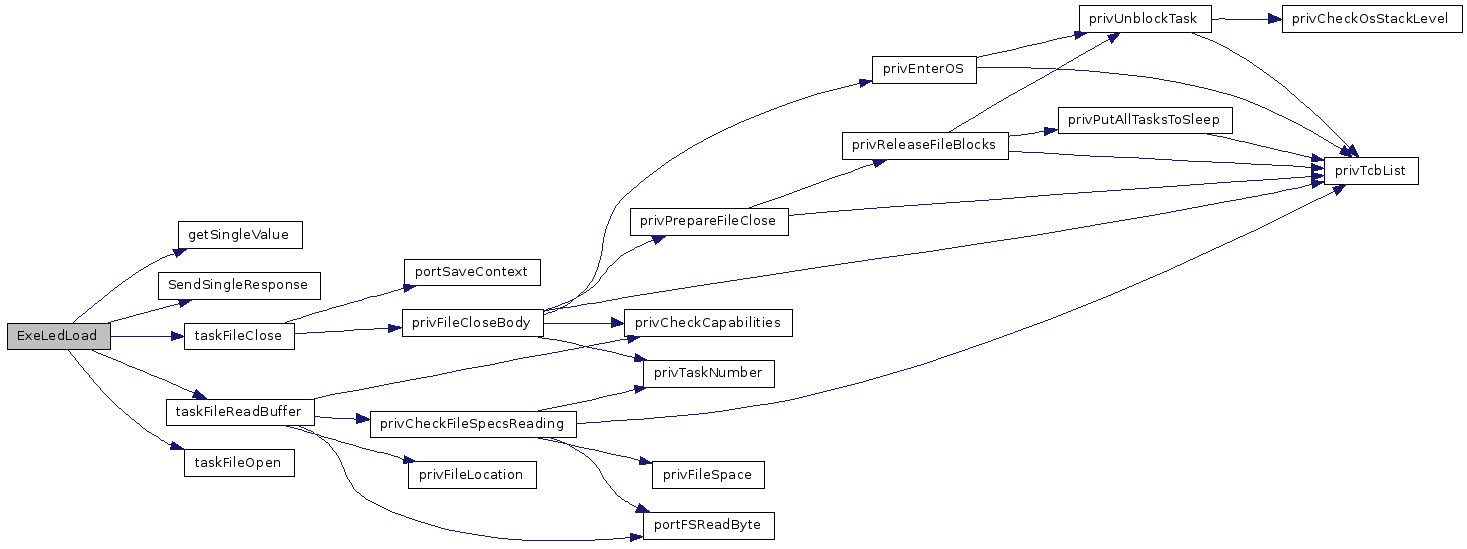Here is the caller graph for this function: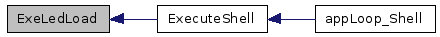static void ExeLedSave ( ) ` [static]`

Definition at line 421 of file code_TestShell.c.

Referenced by ExecuteShell().

Here is the call graph for this function: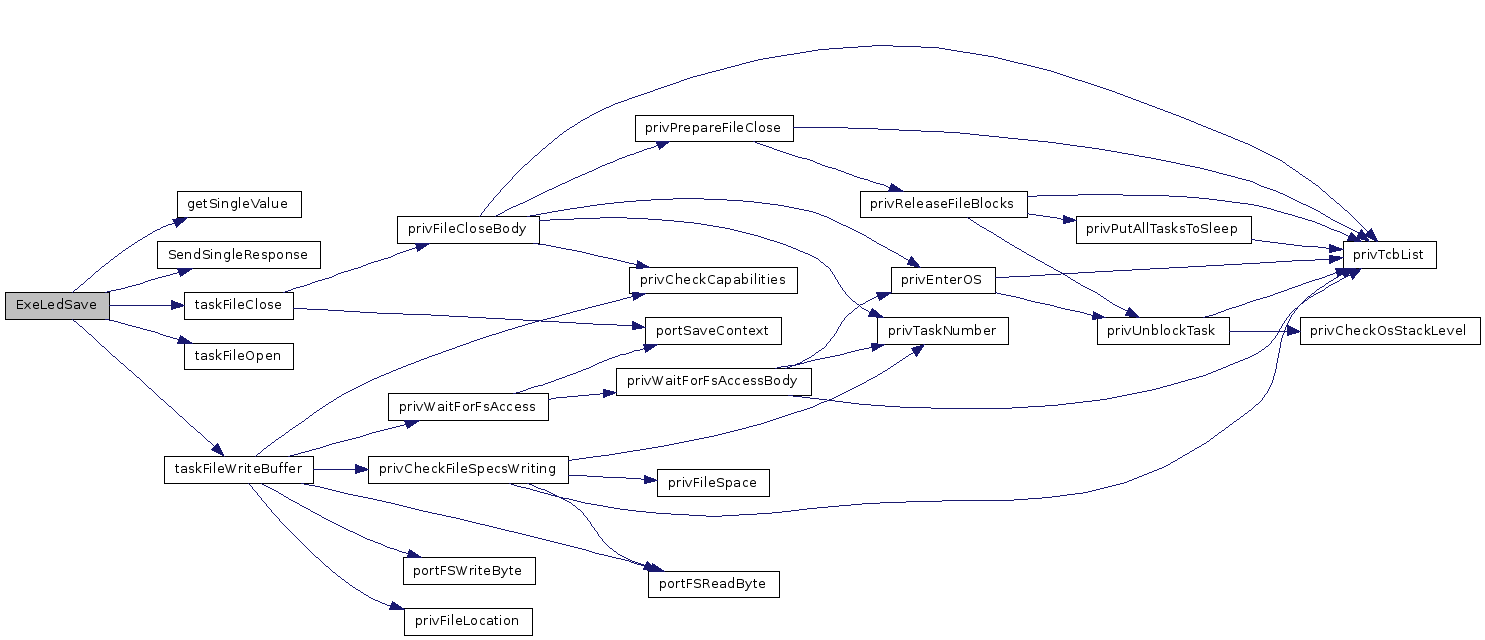Here is the caller graph for this function: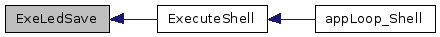static void ExeLedState ( ) ` [static]`

Definition at line 384 of file code_TestShell.c.

Referenced by ExecuteShell().

Here is the call graph for this function:Here is the caller graph for this function:static bit getACK ( ) ` [static]`

Definition at line 100 of file code_TestShell.c.

References GETBIT.

Referenced by startCommunication(), startWrite(), and writeByte().

Here is the caller graph for this function: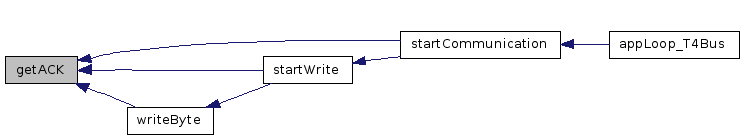static Tbyte getCRC ( ) ` [static]`

Definition at line 111 of file code_TestShell.c.

Referenced by startWrite().

Here is the caller graph for this function: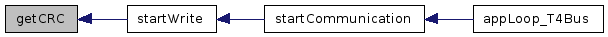static bit getSCLin ( ) ` [static]`

Definition at line 90 of file code_TestShell.c.

References GETBIT.

Here is the caller graph for this function:static bit getSDA ( ) ` [static]`

Definition at line 99 of file code_TestShell.c.

References GETBIT.

Here is the caller graph for this function: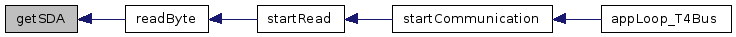static Tuint08 getSingleValue ( ) ` [static]`

Definition at line 257 of file code_TestShell.c.

Here is the caller graph for this function:static void HandleCommand ( void(*)(Tuint08 uiTN) pCommand ) ` [static]`

Definition at line 277 of file code_TestShell.c.

Referenced by ExecuteShell().

Here is the call graph for this function: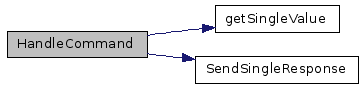Here is the caller graph for this function: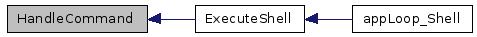static void HandleDump ( ) ` [static]`

Definition at line 295 of file code_TestShell.c.

Referenced by ExecuteShell().

Here is the call graph for this function: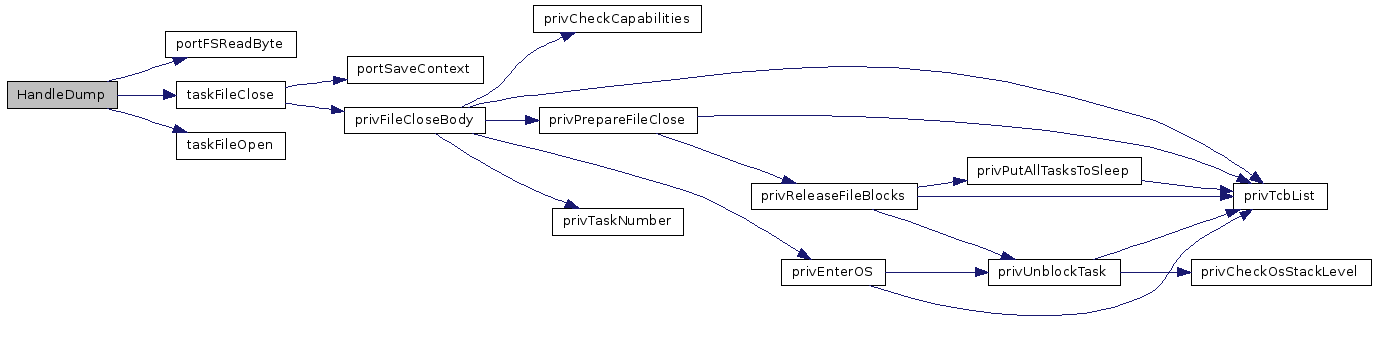Here is the caller graph for this function: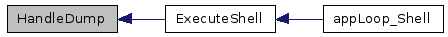static void HandlePriority ( ) ` [static]`

Definition at line 285 of file code_TestShell.c.

Referenced by ExecuteShell().

Here is the call graph for this function: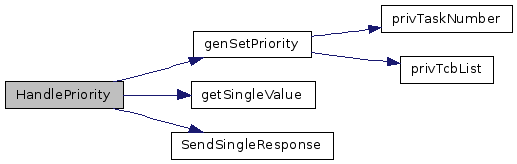Here is the caller graph for this function: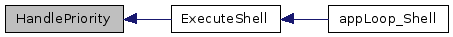static void HandleReboot ( ) ` [static]`

Definition at line 263 of file code_TestShell.c.

References defResponseOK, genReboot(), SendSingleResponse(), and taskDelayFromNow().

Referenced by ExecuteShell().

Here is the call graph for this function: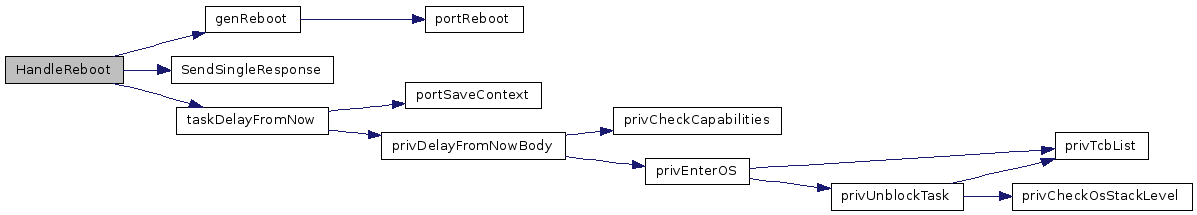Here is the caller graph for this function: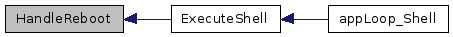static void HandleRecall ( ) ` [static]`

Definition at line 352 of file code_TestShell.c.

Referenced by ExecuteShell().

Here is the call graph for this function:Here is the caller graph for this function: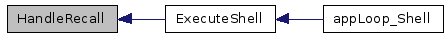static void HandleRecreate ( ) ` [static]`

Definition at line 268 of file code_TestShell.c.

Referenced by ExecuteShell().

Here is the call graph for this function: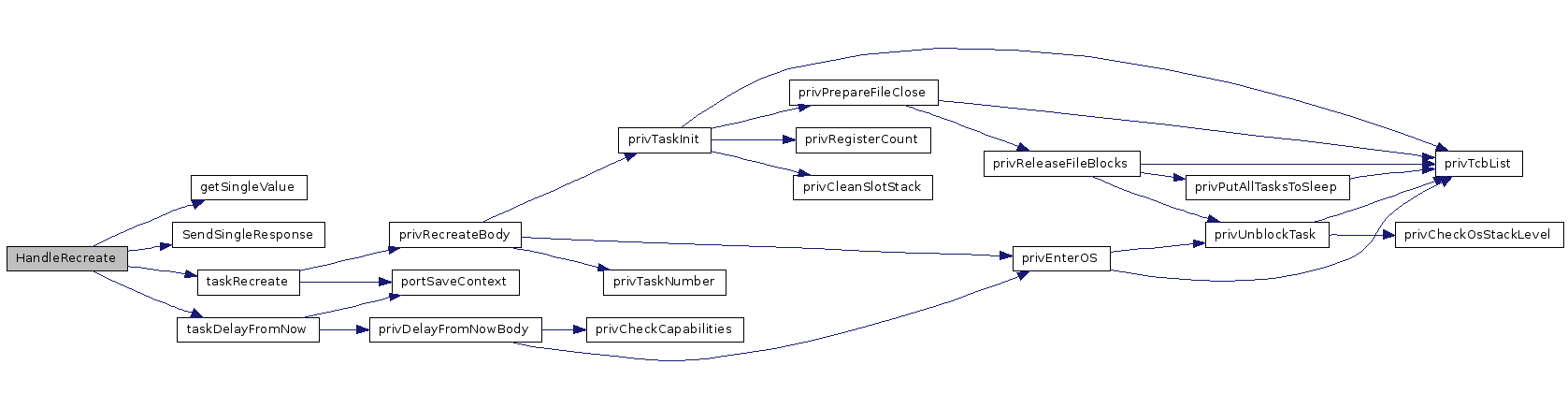Here is the caller graph for this function: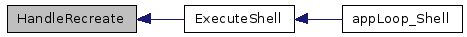static void HandleStore ( ) ` [static]`

Definition at line 328 of file code_TestShell.c.

Referenced by ExecuteShell().

Here is the call graph for this function: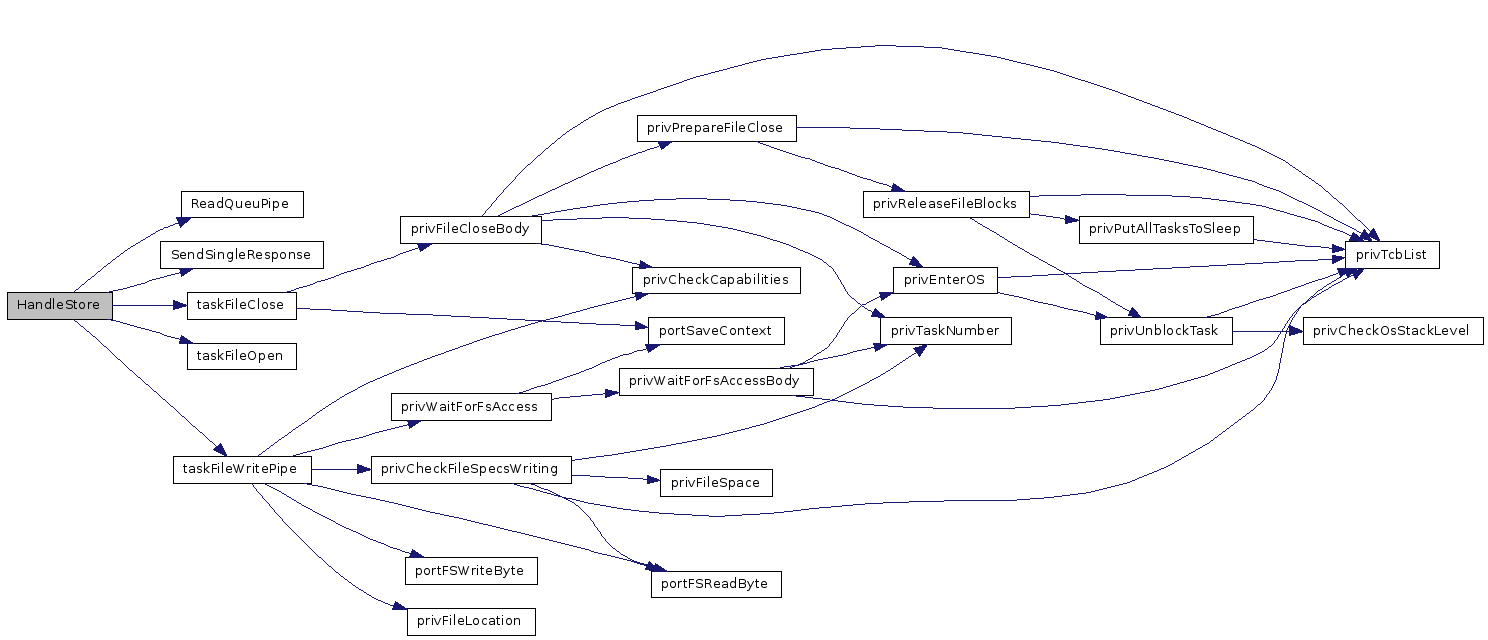Here is the caller graph for this function: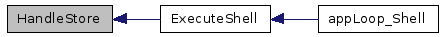static void HandleTest ( ) ` [static]`

Definition at line 376 of file code_TestShell.c.

References defResponseOK, and SendSingleResponse().

Referenced by ExecuteShell().

Here is the call graph for this function:Here is the caller graph for this function:static void initBus ( void ) ` [static]`

Definition at line 188 of file code_TestShell.c.

References initPins(), prevMaster, and virgin.

Referenced by appLoop_T4Bus().

Here is the call graph for this function: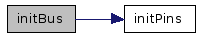Here is the caller graph for this function: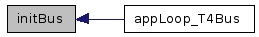static void initPins ( ) ` [static]`

Definition at line 121 of file code_TestShell.c.

Referenced by initBus().

Here is the caller graph for this function: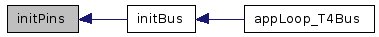static void propDelay ( ) ` [static]`

Definition at line 107 of file code_TestShell.c.

Referenced by startCommunication().

Here is the caller graph for this function:static Tbyte readByte ( ) ` [static]`

Definition at line 139 of file code_TestShell.c.

References getSCLin(), getSDA(), setACK(), Tbyte, and Tuint08.

Here is the call graph for this function: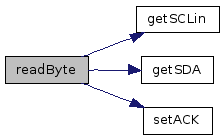Here is the caller graph for this function: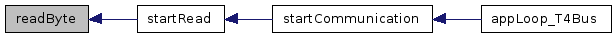static Tchar ReadQueuPipe ( ) ` [static]`

Definition at line 217 of file code_TestShell.c.

Referenced by HandleStore().

Here is the caller graph for this function: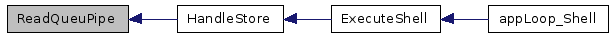static void SendID ( ) ` [static]`

Definition at line 221 of file code_TestShell.c.

Referenced by ExecuteShell().

Here is the call graph for this function:Here is the caller graph for this function: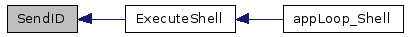static void SendProcessList ( ) ` [static]`

Definition at line 242 of file code_TestShell.c.

Referenced by ExecuteShell().

Here is the call graph for this function:Here is the caller graph for this function: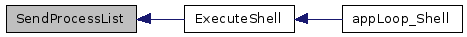static void SendSingleResponse ( Tuint08 uiResponse ) ` [static]`

Definition at line 252 of file code_TestShell.c.

Here is the caller graph for this function: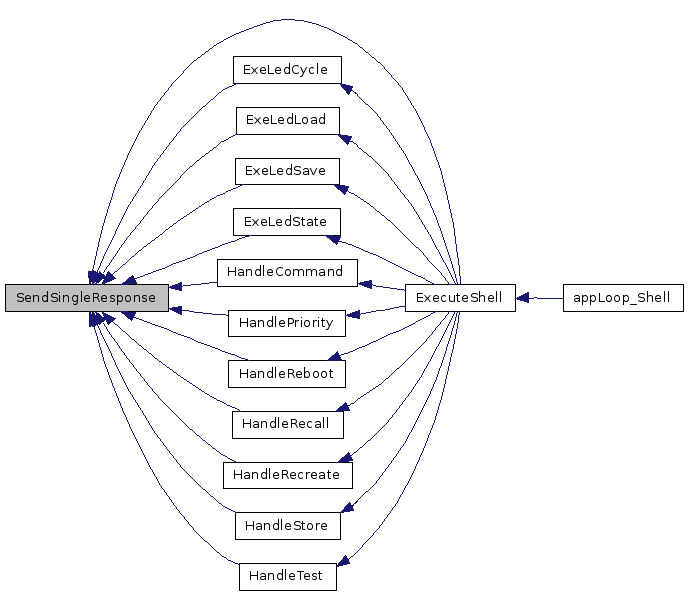static void SendTickCount ( ) ` [static]`

Definition at line 227 of file code_TestShell.c.

Referenced by ExecuteShell().

Here is the call graph for this function: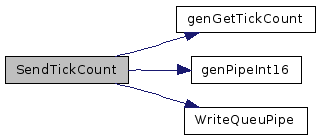Here is the caller graph for this function: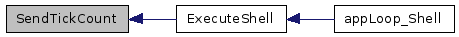static void SendUptime ( ) ` [static]`

Definition at line 233 of file code_TestShell.c.

Referenced by ExecuteShell().

Here is the call graph for this function:Here is the caller graph for this function:static void setACK ( bit b ) ` [static]`

Definition at line 104 of file code_TestShell.c.

References SETBIT.

Here is the caller graph for this function: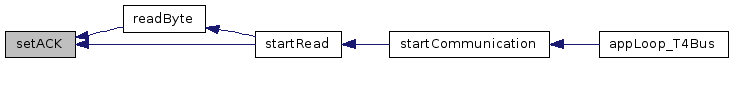static void setSCLout ( bit b ) ` [static]`

Definition at line 102 of file code_TestShell.c.

References SETBIT.

Referenced by startCommunication(), startWrite(), and writeByte().

Here is the caller graph for this function:static void setSDA ( bit b ) ` [static]`

Definition at line 103 of file code_TestShell.c.

References SETBIT.

Referenced by startWrite(), and writeByte().

Here is the caller graph for this function: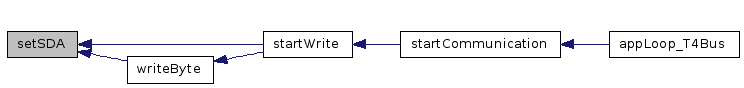void SIG_PIN_CHANGE ( void )

Definition at line 562 of file code_TestShell.c.

 static void startCommunication ( void ) ` [static]`

Definition at line 193 of file code_TestShell.c.

Referenced by appLoop_T4Bus().

Here is the call graph for this function: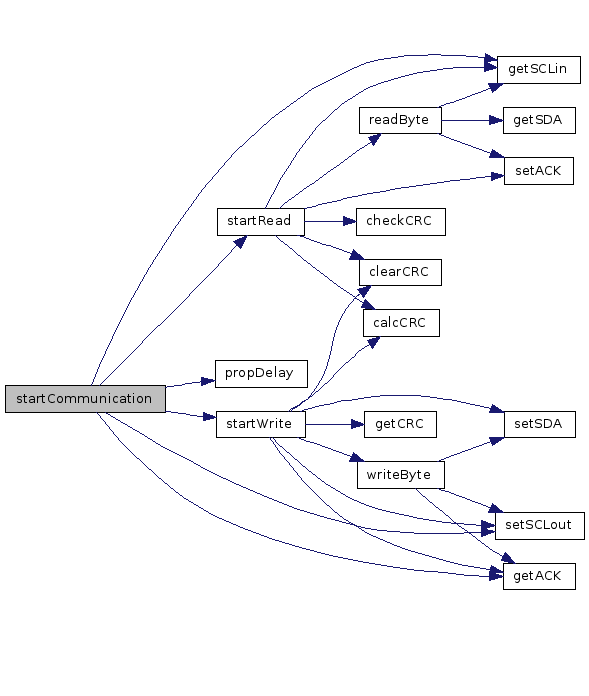Here is the caller graph for this function: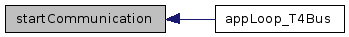static void startRead ( ) ` [static]`

Definition at line 151 of file code_TestShell.c.

Referenced by startCommunication().

Here is the call graph for this function:Here is the caller graph for this function: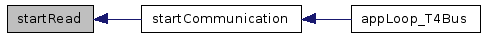static void startWrite ( ) ` [static]`

Definition at line 170 of file code_TestShell.c.

Referenced by startCommunication().

Here is the call graph for this function:Here is the caller graph for this function: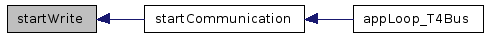static void wait ( Tuint08 uiLed ) ` [static]`

Definition at line 538 of file code_TestShell.c.

Here is the call graph for this function:Here is the caller graph for this function: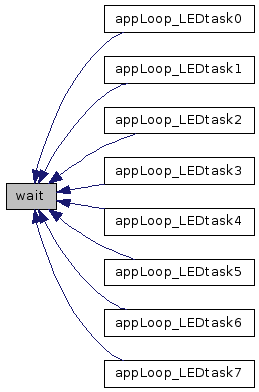static void writeByte ( Tbyte b ) ` [static]`

Definition at line 129 of file code_TestShell.c.

References getACK(), setSCLout(), setSDA(), and Tuint08.

Referenced by startWrite().

Here is the call graph for this function: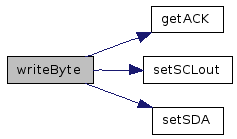Here is the caller graph for this function: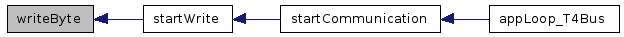static void WriteQueuPipe ( Tchar c ) ` [static]`

Definition at line 216 of file code_TestShell.c.

References genQueuWriteOnName.

Referenced by HandleRecall(), SendID(), SendProcessList(), SendTickCount(), and SendUptime().

Here is the caller graph for this function: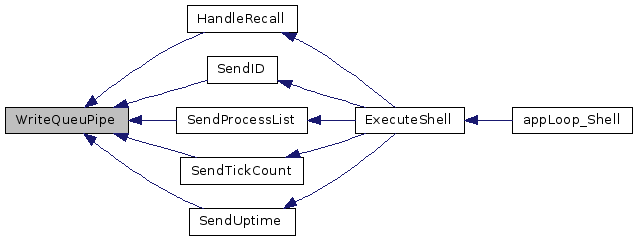## Variable Documentation

 Tbool crcCheckResult` [static]`

Definition at line 77 of file code_TestShell.c.

 Tbyte crcValue` [static]`

Definition at line 74 of file code_TestShell.c.

 Tbool prevMaster` [static]`

Definition at line 76 of file code_TestShell.c.

Referenced by initBus(), startCommunication(), startRead(), and startWrite().

 const Tchar FemtoOSid [] PROGMEM = SystemID

Definition at line 219 of file code_TestShell.c.

 Tuint08 volatile uiLedState` [static]`

Definition at line 380 of file code_TestShell.c.

 Tuint08 volatile uiPeriodOff` [static]`

Definition at line 381 of file code_TestShell.c.

 Tuint08 volatile uiPeriodOn` [static]`

Definition at line 382 of file code_TestShell.c.

 Tuint08 uptime` [static]`

Definition at line 78 of file code_TestShell.c.

Referenced by appTick16(), and SendUptime().

 Tbool virgin` [static]`

Definition at line 75 of file code_TestShell.c.

Referenced by initBus(), startCommunication(), startRead(), and startWrite().

Generated on Fri Oct 16 00:05:27 2009 for FemtoOS by1.5.2